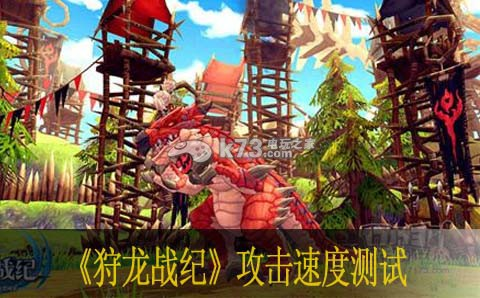# 狩龙战纪攻击速度测试0+25 =>5.0%      √25=5 天赋+杂七杂八

0+35 =>5.9%      √35=5.916 天赋+杂七杂八

0+900 =>30%     √900=30 buff

0+925 =>30.4%   √925=30.41 buff+天赋+杂七杂八

0+935 =>30.6%   √935=30.577 buff+天赋+杂七杂八

0+1835 =>42.8% √1835=42.83 buff+无尽怒火+天赋+杂七杂八

验证码：### 相关导航83k73手游